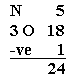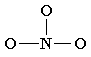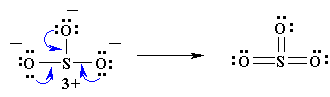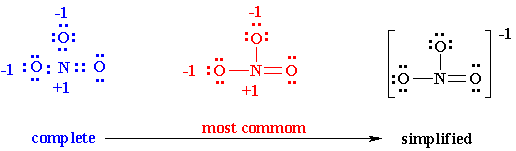Chapter 1: Chemical BondingHow to draw Lewis Diagrams

An outline of how to detemine the "best" Lewis structure for an example, NO3- is given below:

1.  Determine the total number of valence electrons in a molecule2.  Draw a skeleton for the molecule which connects all atoms using only single bonds.  In simple molecules, the atom with the most available sites for bondng is usually placed central.  The number of bonding sites is detemined by considering the number of valence electrons and the ability of an atom to expand it's octet.  As you become better, you will be able to recognise that certain groups of atoms prefer to bond together in a certain way.3.  Of the 24 valence electrons in NO3-, 6 were required to make the skeleton. Consider the remaining 18 electrons and place them so as to fill the ocets of as many atoms as possible (start with the most electronegative atoms first then proceed to the more electropositive atoms).4.  Are the octets of all the atoms filled?   If not then fill the remaining octets by making multiple bonds (make a lone pair of electrons, located on a more electronegative atom, into a bonding pair of electrons that is shared with the atom that is electron defficient).5.  Check that you have the lowest FORMAL CHARGES possible for all the atoms, without violating the octet rule;       (valence e-) - (1/2 bonding e-) - (lone electrons).IMPORTANT : no Lewis diagram is complete without formal charges.  Lewis diagrams are drawn to examine mechanisms so knowing which parts of a molecule are electron defficient (+) and which are electron rich (-) is vital.
It is best to have a formal charge of 0 for as many of the atoms in a structure as possible.
If a formal charge of 1- is located next to a formal charge of 1+, the formal charges can usually be minimized by having a lone pair of electrons, located on the atom with the 1- charge become a bonding pair of electrons that is shared with the atom that has the 1+ formal charge (this can be visualised in the same way as the formation of multiple bonds were above).

CAUTION : octets can be expanded to minimise formal charges but only for atoms in the second row of the periodic table  (where n=3 or greater).   For instance in our example, N cannot expand its octet so keeps a formal charge of 1+ and both singly bonded oxygens a formal charge of 1-.  If our molecule were SO3 , however, it would be possible to minimize all formal charges by having the sulfur expand its octet.6.  You may find that the best Lewis diagram (the one with the lowest formal charges and all octets satisfied) is given in a number of different ways.  For NO3-, three different diagrams are given below.  From left to right they start with the most complete Lewis diagram to the most simplified.Why so many different ways?  Depends on the need of the chemist. For instance, complete structures are more useful for the novice organic chemist learning to appreciate the mechanism of a reaction while simplified versions may be preferred by inorganic chemists.

Now you should be ready to try some questions.© Dr. Ian Hunt, Department of Chemistry, University of Calgary